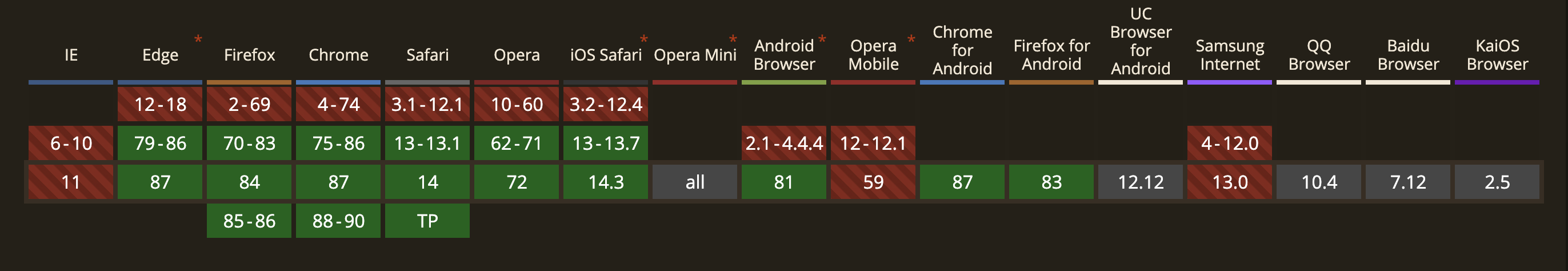# Numeric Separators in JavaScript

Numeric Separators give us the ability to separate thousands with an underscore (_) in numeric literals.

### How it’s useful❓

`let series = 10000;`

Numeric Separators in javascript, enables underscore as a separator in numeric literals to improve readability.

Example:

`let series = 1_00_00;`

You can also use this for binary, octal, and hex numbers.

Binary Number 👻
`let series1 = 0b1010_0101_1001;console.log(series1); // 2649`
Octal Number: 👻
`let series2 = 0o2_3_5_7;console.log(series2); // 1263`
Hex Number: 👻
`let series3 = 0xA_B_C_D_E;console.log(series3); // 703710`

### Few Limitation 🤦‍♀️

Below limitation snippet will throw SyntaxError

1. More than one underscore in a row is not allowed

`let series1 = 100__000;`
2. Can not be used after leading 0

`let series2 = 0_1;`
3. Not allowed at the end of numeric literals

`let series3= 100_;`

### Browser Support 🎗